Journal of Integer Sequences, Vol. 16 (2013), Article 13.1.2

## Can the Arithmetic Derivative be Defined on a Non-Unique Factorization Domain?

### Pentti Haukkanen, Mika Mattila, and Jorma K. Merikoski School of Information Sciences FI-33014 University of Tampere Finland Timo Tossavainen School of Applied Educational Science and Teacher Education University of Eastern Finland P.O.Box 86, FI-57101 Savonlinna Finland

Abstract:

Given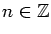, its arithmetic derivative n' is defined as follows: (i)  0'=1'=(-1)'=0. (ii) If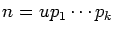, where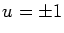and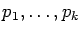are primes (some of them possibly equal), then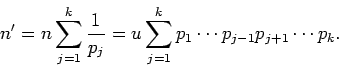An analogous definition can be given in any unique factorization domain. What about the converse? Can the arithmetic derivative be (well-)defined on a non-unique factorization domain? In the general case, this remains to be seen, but we answer the question negatively for the integers of certain quadratic fields. We also give a sufficient condition under which the answer is negative.

Full version:  pdf,    dvi,    ps,    latex

(Concerned with sequences A000040 A003415 A005117.)

Received October 30 2012; revised version received January 1 2013. Published in Journal of Integer Sequences, January 1 2013.